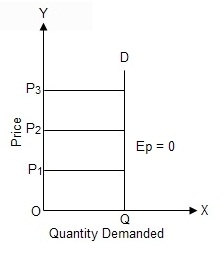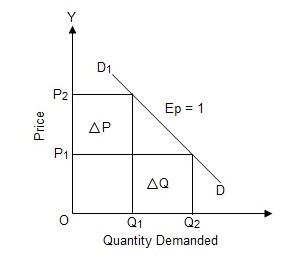Types of Price Elasticity of DemandTypes/Degree  of Price Demand

1.       Perfectly Elastic Demand
When negligible change in price leads to infinite change in quantity demanded ,the demand for a commodity is said to be perfectly elastic demand.In the given figure, the price is measured in OY-axis and quantity demanded is measured along the OX-axis. PD is the demand curve which is parallel to OX-axis. Perfectly elastic demand shows that quantity demanded for a goods increases infinitely with a small fall in price or becomes zero with a small rise in price.

2.       Perfectly Inelastic Demand(Ep=0)
When the quantity demanded does not change with the change in its price, the demand for a commodity is said to perfectly inelastic demand.In the given figure, the price is measured in OY-axis and quantity demanded is measured along the OX-axis. QD is the demand curve which is parallel to OY-axis. Here, whatever be the price either OP1 or OP2 or OP quantity demanded is same as OQ.

3.       Unitary Elastic Demand: (Ep=1)
When the percentage change in quantity demanded is equal to the percentage change in price of  commodity it is said to be unitary elastic demand.In the given figure, the price is measured along OY-axis and quantity demanded is measured along the OX-axis. D1D is the demand curve which is unitary elastic, Here, the increase in quantity demanded for a goods from OQto OQ2 equal to the decrease in price of goods from OPto OP1. So, it indicates unitary elastic demand.

4.       Relatively Elastic Demand (Ep>1)
When the percentage change in the quantity  demanded for a commodity is more than percentage change in its price, it is called relatively elastic demand.In the given figure, the price is measured along OY-axis

and quantity demanded is measured along OX-axis. D1D is the demand curve which is relatively elastic. Here, increase in quantity demanded from OQ1 to OQ2 is more than the decrease in price from OP2 to OP1. Thus, it indicates relatively elastic demand.

5. Relatively Inelastic Demand (Ep<1)

When the percentage change in the quantity demanded of a commodity is less than the percentage change in its price, it is called relatively inelastic demand.In the given figure, the price is measured along OY-axis and quantity demanded is measured along the OX-axis. D1D is the demand curve which is relatively inelastic. Here, the increase in quantity demanded for a goods from OQto OQis less than the decreases in price of the goods from OP2 to OP1. So, it indicates relatively inelastic demand.